# 3-D prestack depth migration of common-azimuth data

Biondo Biondi and Gopal Palacharla

biondo@sep.stanford.edu, gopal@sep.stanford.edu

# ABSTRACT

We extended the common-azimuth 3-D prestack migration method presented in a previous report () to the important case of imaging common-azimuth data when the velocity field varies laterally. This generalization leads to an efficient mixed space-wavenumber domain algorithm for 3-D prestack depth migration of common-azimuth data. We implemented the method using a split-step scheme, and applied it to the migration of two synthetic data sets: the first data set was generated assuming a vertical velocity gradient, the second one assuming a velocity gradient with both a vertical and a horizontal component. Common-azimuth migration correctly imaged the reflectors in both cases.

INTRODUCTION

For 3-D prestack migration, Kirchhoff methods are usually preferred over methods based on recursive downward continuation because of their flexibility in handling 3-D prestack data geometries (). Kirchhoff methods can be employed to efficiently migrate data sets with uneven spatial sampling and data sets that are subsets of the complete prestack data, such as common-offset cubes and common-azimuth cubes. For recursive methods the irregular sampling problem can be addressed with an interpolation preprocessing step, though in practice it can be a challenging task. However, in principle the downward continuation of prestack subsets should be carried out in the full 5-D data space, even when the original subset is 3-D (common offset) or 4-D (common azimuth). As a result of these constraints on the dimensionality of the computational domain, most of the computations are wasted on propagating components of the wavefield that either are equal to zero or do not contribute to the final image. These potential limitations of recursive methods have led the industry to adopt almost exclusively Kirchhoff methods for 3-D prestack migration (, ), though recursive methods have some intrinsic advantages over Kirchhoff methods. First, they are potentially more accurate and robust because they are based on the full wave-equation and not on an asymptotic solution based on ray theory. Second, when they can be used to extrapolate the recorded data without increasing their dimensionality (e.g. zero-offset data), they can be implemented more efficiently than the corresponding Kirchhoff methods.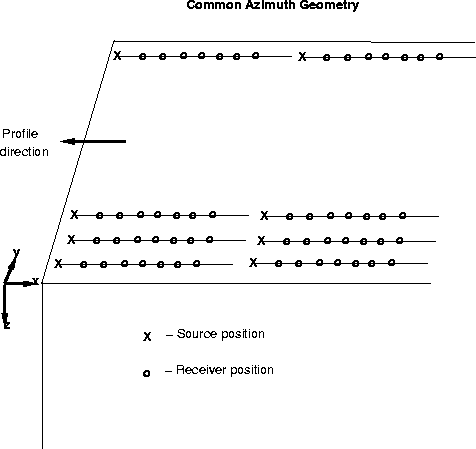acquis
Figure 1
Schematic showing the acquisition geometry of common-azimuth data. The data are acquired along parallel lines, with all the offset vectors aligned along a common azimuth. For simplicity, but without loss of generality, we assume that the common offset-azimuth is aligned along the in-line direction.In a previous report () we introduced a method for efficiently migrating common-azimuth data (see Figurefor a schematic representation of common-azimuth acquisition geometry) by recursive downward continuation in the original 4-D space. An efficient algorithm for migrating common-azimuth subsets has useful practical applications because common-azimuth data are either the result of a collection of actual physical experiments (e.g. single-streamer marine survey with negligible cable feather) or they may be synthesized by preprocessing (). However, in the previous report we applied common-azimuth migration to the case of limited interest when velocity varies only with depth; we did not address the important issue of applying the method to the general case when the velocity function is laterally varying. Similarly, Ikelle developed (unknown to us at the time we wrote the first report) a linearized inversion method for common-azimuth data that shares with our previous work the same theoretical basis, but also shares the same limitations of applicability to a medium with only vertical velocity variations. In this paper, we overcome these limitations and we generalize common-azimuth migration to the case of laterally varying velocity.

The generalization of common-azimuth migration presented in this paper is based on two new theoretical insights. First, we derive common-azimuth migration as a recursive downward continuation by a new operator that continues common-azimuth data recorded at one depth level to common-azimuth data recorded at the next depth level. The previous derivation of common-azimuth migration was based on stationary-phase approximation to the whole process of downward continuation followed by imaging at zero offset. Isolating the common-azimuth downward continuation from imaging enables us to better understand the assumptions underlying common-azimuth migration. The second improvement in the theory follows from a ray-based geometric interpretation of common-azimuth downward continuation. Thanks to this ray interpretation we derived and justified a more general formulation of the stationary-phase result that can be applied to downward continuation in presence of lateral velocity variations. Furthermore, the geometric insight enables us to analyze the errors caused by the use of common-azimuth continuation in place of full 3-D prestack continuation and to gain an appreciation of the limitations and strengths of the proposed method.

COMMON-AZIMUTH DOWNWARD CONTINUATION We first derive a wave-equation operator for downward continuing common-azimuth data by applying a stationary phase approximation to the frequency-wavenumber domain formulation of the full 3-D prestack downward continuation operator. Then, we present a ray-based geometric interpretation of the stationary-phase result, and use this geometric interpretation to generalize the frequency-wavenumber results to a medium where the velocity function varies laterally, and to analyze the errors caused by the use of common-azimuth continuation in the presence of lateral velocity variations.

The acquisition geometry of common-azimuth data is shown in Figure. Without loss of generality we assume that the common offset-azimuth is aligned with the x axis (in-line axis). Since the offset vectors between source and receivers are constrained to have the same azimuth, common-azimuth data has only four dimensions. The four axes are: recording time, two midpoints, and the offset along the azimuthal direction. A common azimuth data set can be downward continued by applying the full 3-D prestack downward continuation operator, that in the frequency-wavenumber domain can be expressed as a phase-shift operator, with the phase given by the 3-D double square root equation (DSR). In 3-D the DSR equation is a function of five scalar variables: the temporal frequency (), the two components of the midpoint wavenumber vector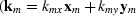), and the two components of the offset wavenumber evaluation of the phase function). The downward continuation of common-azimuthdata from depth z to depth z+ dz by full 3-D prestack continuation can be expressed as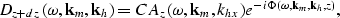(1)
where the phase function is given by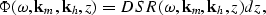(2)
and the double-square root equation is equal to(3)
whereand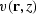are the velocities evaluated respectively at the source and receiver locations at depth z. The distinction between velocity at the source and velocity at the receiver may seem somewhat artificial in the wavenumber domain, but it is important to keep them as two separate parameters during the stationary-phase approximation to derive a method applicable to depth migration. A physical interpretation of the significance of the source and receiver velocity terms is possible after the geometric interpretation of the stationary-phase results presented in the next section.

The result of the downward continuation with the full 3-D prestack is five dimensional, although the original common-azimuth data is only four dimensional. This increase in the dimensionality of the computational domain causes a drastic increase in the computational and memory requirements of 3-D prestack downward continuation, making it unattractive compared with other migration methods, such as the ones based on the Kirchhoff integral. However, the computational cost can be greatly reduced by applying a new downward continuation operator that evaluates the wavefield at the new depth level only along the offset-azimuth of the original data. We derive this operator by evaluating the data at the new depth level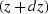at the origin of the cross-line offset axis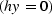by integration over the cross-line offset wavenumber,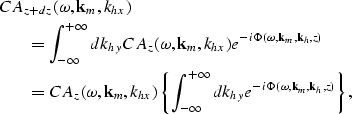(4)
and by recognizing that since common-azimuth data is independent of khy the integral can be pulled inside and analytically approximated by the stationary phase method (). The common-azimuth downward continuation operator can thus be written as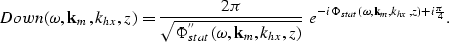(5)
The phase function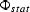is equal to the phase function in equation (2) evaluated along the stationary path, and is equal to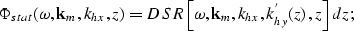(6)
where and khy'(z) is the stationary path for the double-square root equation, as a function of khy. There are two solutions for the stationary path of the double-square root equation; they are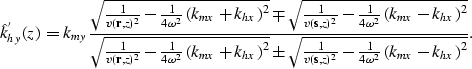(7)
In choosing between these two solutions, we first notice that one solution is the inverse of the other. Second we consider the limiting case of the in-line offset wavenumber (khx) equal to zero. In this case one solution diverges while the other (being the one with the minus sign at the numerator) is equal to zero. We accept this second solution because it is consistent with the notion that when both khx and khy vanish the double square root equation reduces to the single square root equation that is commonly used for migrating zero-offset data ().

The implementation of common-azimuth downward continuation as described by equation (3) through equation (7) yields an algorithm for efficient migration of common-azimuth data. When the velocity field is only a function of depth the method can be efficiently implemented by a simple phase-shift algorithm. When the velocity field is also function of the lateral coordinates, and accurate depth-migration is required, the method should be implemented using a mixed space-wavenumber domain migration scheme, such as Phase-Shift Plus Interpolation (PSPI) () or split step (). We implemented the equations using a straightforward generalization of split step to prestack migration because of its simplicity and computational efficiency; other choices would have probably been just as effective.

In the next two sections, we analyze common-azimuth downward continuation by using a ray-theoretical interpretation of the method. The goal is to understand the underlying assumptions, and to understand the accuracy limitation of the method when velocity variations cause ray bending.